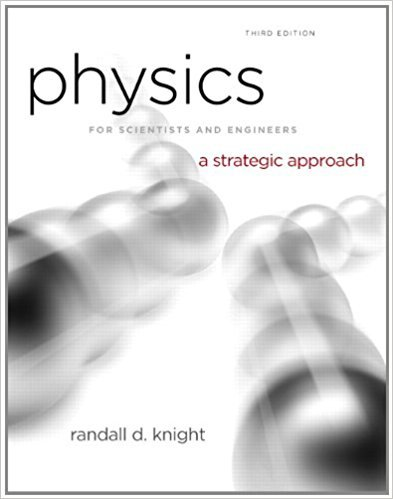×
Get Full Access to Physics For Scientists And Engineers: A Strategic Approach With Modern Physics - 3 Edition - Chapter 17 - Problem 51p
Get Full Access to Physics For Scientists And Engineers: A Strategic Approach With Modern Physics - 3 Edition - Chapter 17 - Problem 51p

×

# A 6.0-cm-diameter cylinder of nitrogen gas has aISBN: 9780321740908 69

## Solution for problem 51P Chapter 17

Physics for Scientists and Engineers: A Strategic Approach with Modern Physics | 3rd Edition

• Textbook Solutions
• 2901 Step-by-step solutions solved by professors and subject experts
• Get 24/7 help from StudySoup virtual teaching assistantsPhysics for Scientists and Engineers: A Strategic Approach with Modern Physics | 3rd Edition

4 5 1 410 Reviews
24
5
Problem 51P

Problem 51P

A 6.0-cm-diameter cylinder of nitrogen gas has a 4.0-cm-thick movable copper piston. The cylinder is oriented vertically, as shown in FIGURE, and the air above the piston is evacuated. When the gas temperature is 20°C, the piston floats 20 cm above the bottom of the cylinder.

a. What is the gas pressure?

b. How many gas molecules are in the cylinder?

Then 2.0 J of heat energy are transferred to the gas.

c. What is the new equilibrium temperature of the gas?

d. What is the final height of the piston?

e. How much work is done on the gas as the piston rises?

FIGUREStep-by-Step Solution:

Solution 51 P:

Step 1 of az:-

a)

Here we have to find the gas pressure.

The diameter of the cylinder is,..

The piston is made up of copper.

The thickness of the piston is 4 cm or 0.04 m.

First of all we have to find the mass of the piston and it can be calculated as,Whereis the density of copper and V is the volume of the piston.

So,The pressure will be,The density of copper is,.

So, the gas pressure must be equal to 3.512 kPa.

b)

Here we have to find the number of gas molecules in the cylinder.

We can apply the ideal gas equation by some approximation.

So,Here R’ is the specific gas constant for nitrogen.So, the number of molecules inside can be calculated as,.

So, the number of molecules will be,So, there will bemolecules inside the cylinder, when the temperature isC.

c)

Here we have to calculate the equilibrium temperature of the gas.

Inside the gas is nitrogen.

The molar mass ofmolecules is 28 g/mol.

We know that,Step 2 of 1

##### ISBN: 9780321740908

Unlock Textbook Solution